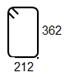# Bar Bending Schedule for Reinforced Concrete BeamReading time: 1 minute

Bar bending schedule provides the reinforcement calculation for reinforced concrete beam. It provides details of reinforcement cutting length, type of bends and bend length. We will take one example for reinforcement quantity calculations for a concrete beam.

## Bar Bending Schedule for Reinforced Concrete Beam

### Example of Beam Reinforcement Calculation:

Consider a beam of clear length of 4m, 300mm wide by 450mm depth. It consists of 2-12 diameter bars at top, and 2-16 diameter and 1 - 12 diameter bars at the bottom. Diameter of stirrup is 8mm spaced at 180mm center to center. Clear cover to reinforcement provided is 40mm.Fig: RCC Beam Reinforcement DetailsFig: RCC Beam Cross-Section

Now we will calculate the length of reinforcement based on shapes of reinforcement required for reinforced concrete beam in above example. We will start with bottom reinforcement, B1. Bar shape of B1 is as shown below: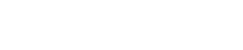Length of B1 = clear distance between walls + 2 x width of walls - 2 x bar cover + 2 x bend length Bend length = 6 x 16 = 96 consider as 100mm Bend length is calculated as 6 x diameter of bar for reinforcement conforming to IS: 1786-1961 Length of B1 = 4000 + 2 x 230 - 2 x 40 + 2 x100 = 4580mm Length of bar B2 is calculated based on shape of this bar. This bar bends up near the support as shown below: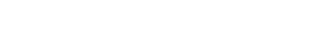Length of bar B2: A + B + C = 4000 + 2 x 230 - 2 x 40 + (1.414xH - H) H = 450 - 2 x 40 - 2 x 12 - 2 x 12/2 = 334mm B2 = 4000 + 2 x 230 - 2 x 40 + (1.414x334 - 334) = 4518.3 = 4520mm Length of Bar T1 = 4000 + 2 x 230 -2 x 40 = 4380mm Length of Stirrups S1:Stirrups are spaced at 180mm center to center. Stirrups are provided between walls or support for a beam. No. of stirrups required for given beam =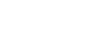Length a = 450 - 2 x40 - 8 = 362mm Length b = 300 - 2 x 40 - 8 = 212mm Therefore, length of 1 stirrup S1 = 2 x (212 + 362 + 90) = 1328 mm Where 90mm is the minimum hook length as per IS 2502 - Table - II.

## Bar Bending Schedule for RCC Beam:

 No. Bar Mark Bar dia (mm) No. of bars Length (mm) Weight of bars (kg) Bar Shape 1 B1 16 2 4580 14.5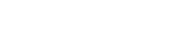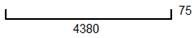2 B2 12 1 4520 4.02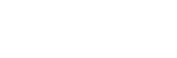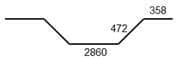3 T1 12 2 4380 7.80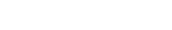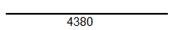4 S1 8 24 1330 12.6